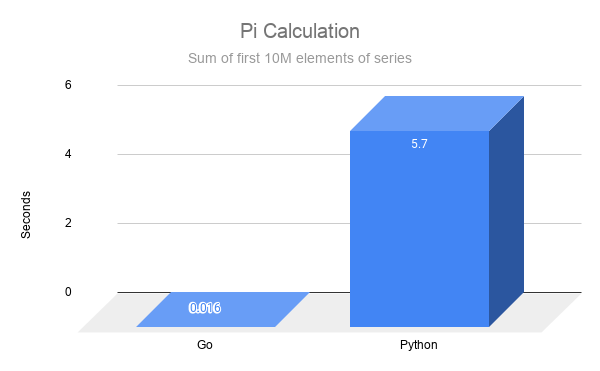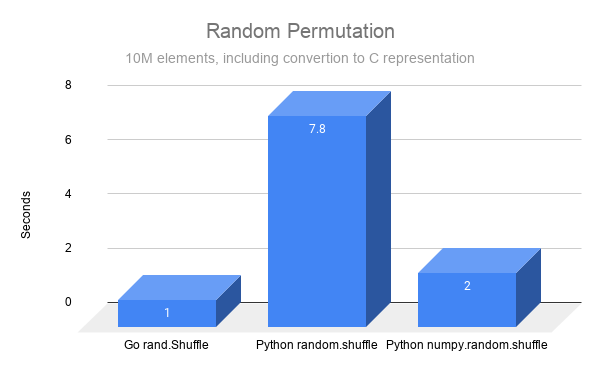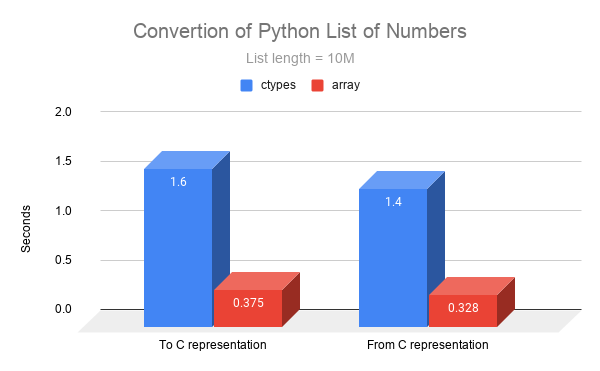Snake + Gopher = <3

By Amit Lavon

# Introduction

When working in Python, it sometimes makes sense to implement parts of the program in a static, high-performance language. Go can be a great choice for that because it is fast, simple and cross platform.

This tutorial started as a cheatsheet I wrote for myself. In my research I work mostly in python. However, since I work on large amounts of data, I needed a heavy-duty language for some of my more demanding analysis tasks. That’s when I started exploring the possibility of exporting parts of my analysis routines to Go.

In order to implement the advice shown here you will need Python 3, a Go compiler, and GCC (on Windows use MinGW). The code examples are available as runnable files on github.

There exist other ways to cross-call Go from Python, such as extension modules and SWIG. Each has its own pros and cons. I chose ctypes because it has no additional dependencies and it is easy to learn.

This tutorial is based on my own experience, so it might contain suboptimal practices or even mistakes. Your feedback and ideas are welcome!

# Hello World

Let’s start with the bare minimum.

hello.go:

``````package main

import "C"
import "fmt"

//export hello
func hello() {
fmt.Println("Hello world!")
}

func main() {}
``````

Build:

``````# Windows:
go build -o hello.dll -buildmode=c-shared hello.go
# Linux:
go build -o hello.so -buildmode=c-shared hello.go
``````

hello.py:

``````import ctypes

lib = ctypes.CDLL('./hello.dll')  # Or hello.so if on Linux.
hello = lib.hello

hello()
``````

Then run:

``````> python hello.py
Hello world!
>
``````

Congrats!

Let’s break it down:

1. The Go code uses regular Go logic, but exports its function for external use with the `//export` directive.
2. Building with `-buildmode=c-shared` creates a C-style shared library.
3. Python loads the shared library and accesses the exported function.

# Primitive Input and Output

Here we introduce some basic arguments and return values. Let’s start with an example.

add.go:

``````//export add
func add(a, b int64) int64 {
return a + b
}
``````

add.py:

``````lib = ctypes.CDLL('./add.dll')
add = lib.add

# Make python convert its values to C representation.
add.argtypes = [ctypes.c_longlong, ctypes.c_longlong]
add.restype = ctypes.c_longlong

print('10 + 15 =', add(10, 15))
``````

Run:

``````> python add.py
10 + 15 = 25
>
``````

To pass input and receive output to/from a Go function, we need to use ctypes’s `argtypes` and `restype` attributes. They do 2 things:

1. `argtypes` guards the function by checking the arguments before calling the library code.
2. Using these attributes tells Python how to convert the input Python values to ctypes values, and how to convert the output back to a Python value.

You can find the mapping between C types and Go types in the generated `.h` file after you compile your Go code with `-buildmode=c-shared`.

DANGER: Some types change sizes on different architectures. It is generally safer to use sized types (`int64`) here than unsized types (`int`).

# Arrays and Slices

We are now entering the zone of unprotected memory access. While Python and Go are generally memory safe, working with raw pointers could end up in buffer overflows and memory leaks.

Make sure to read this section through to learn how to handle pointers safely.

squares.go:

``````// Returns the squares of the input numbers.
//
//export squares
func squares(numsPtr *float64, outPtr *float64, n int64) {
// The way to wrap a pointer with a Go slice.
nums := (*[1 << 30]float64)(unsafe.Pointer(numsPtr))[:n:n]
out := (*[1 << 30]float64)(unsafe.Pointer(outPtr))[:n:n]

for i, x := range nums {
out[i] = x * x
}
}
``````

squares.py:

``````lib = ctypes.CDLL('./squares.dll')
squares = lib.squares

squares.argtypes = [
ctypes.POINTER(ctypes.c_double),
ctypes.POINTER(ctypes.c_double),
ctypes.c_longlong,
]

# Building buffers from arrays is more efficient than
# (ctypes.c_double * 3)(*[1, 2, 3])
nums = array('d', [1, 2, 3])
nums_ptr = (ctypes.c_double * len(nums)).from_buffer(nums)
out = array('d', (0 for _ in range(len(nums))))
out_ptr = (ctypes.c_double * len(out)).from_buffer(out)

squares(nums_ptr, out_ptr, len(nums))
print('nums:', list(nums))
print('out:', list(out))
``````

Run:

``````> python squares.py
nums: [1.0, 2.0, 3.0]
out: [1.0, 4.0, 9.0]
>
``````

In order to work with lists we need to convert them into C arrays. The way to do it is to create an array using `(ctypes.my_type * my_length)(1, 2, 3 ...)`. The faster way to do it is to use the `array` library as demonstrated above. See the benchmarks section for more info about their performance.

In Go you can cast the C-like pointer to a slice (that still points to Python’s memory), see the demonstration above. This way you can utilize Go’s syntax while working with Python buffers.

Output lists are the tricky part. You cannot return a Go pointer when using CGo, that will result in an error. Instead you can allocate a C pointer from Go using `C.malloc()` and return that. However, that pointer is not garbage-collected so unless you implement a way to deallocate those, you will have a memory leak.

My recommendation for safely returning arrays from Go is to either pre-allocate them in Python and pass them as arguments to Go, or to generate the arrays in Go and wrap them in a safe-finalizing struct (see structs section).

#### Summary of Dangers

• Returning Go pointers to Python. Error.
• Returning C pointers from Go to Python without explicit deallocation. Memory leak.
• Losing the `ctypes` reference while Go code is still running (like when taking `ctypes.addressof` and dumping the pointer object). Possible segmentation fault.

# Strings

Strings work pretty much like arrays in terms of memory management, so everything related to arrays applies here too. Below I discuss some convenience techniques and some pitfalls.

repeat.go:

``````//export repeat
func repeat(s *C.char, n int64, out *C.char, outN int64) *C.char {
// Create a Go buffer around the output buffer.
outBytes := (*[1 << 30]byte)(unsafe.Pointer(out))[:0:outN]
buf := bytes.NewBuffer(outBytes)

var goString string = C.GoString(s) // Copy input to Go memory space.
for i := int64(0); i < n; i++ {
buf.WriteString(goString)
}
buf.WriteByte(0) // Null terminator, important!
return out
}
``````

repeat.py:

``````lib = ctypes.CDLL('./repeat.dll')
repeat = lib.repeat

repeat.argtypes = [
ctypes.c_char_p,
ctypes.c_longlong,
ctypes.c_char_p,
ctypes.c_longlong,
]
repeat.restype = ctypes.c_char_p

# Reusable output buffer.
buf_size = 1000
buf = (ctypes.c_char * buf_size)(*( * buf_size))

result = repeat(b'Badger', 4, buf, buf_size)  # type(result) = bytes
print('Badger * 4 =', result.decode())

result = repeat(b'Snake', 5, buf, buf_size)
print('Snake * 5 =', result.decode())
``````

Run:

``````> python repeat.py
Badger * 4 = BadgerBadgerBadgerBadger
Snake * 5 = SnakeSnakeSnakeSnakeSnake
>
``````

Strings are passed by converting a Python string to a bytes object (typically by calling `encode()`), then to a C-pointer and then to a Go-string.

Using `ctypes.c_char_p` in argtypes makes Python expect a bytes object and convert it to a C `*char`. In restype, it converts the returned `*char` to a bytes object.

In Go you can convert a `*char` to a Go string using `C.GoString`. This copies the data and creates a new string managed by Go in terms of garbage collection. To create a `*char` as a return value, you can call `C.CString`. However, the pointer gets lost unless you keep a reference to it, and then you have a memory leak. To return strings from Go, use the same practices as with arrays.

If the output pointer was given by Python, Go can return it and Python will automatically make a bytes object out of it. See the demonstration above.

#### Summary of Dangers

• Returning a `C.CString` without keeping the reference for future deallocation. Memory leak.
• Not appending a null terminator at the end of the output string. Buffer overflow when converting to Python object.
• Not checking output buffer size in Go. Buffer overflow or truncated output.

## Array of Strings

Passing an array of strings can be done with a snippet by Stack Overflow user habnabit.

join.go:

``````func goStrings(cstrs **C.char) []string {
var result []string
slice := (*[1 << 30]*C.char)(unsafe.Pointer(cstrs))[: 1<<30 : 1<<30]
for i := 0; slice[i] != nil; i++ {
result = append(result, C.GoString(slice[i]))
}
return result
}
``````

join.py:

``````def to_c_str_array(strs: List[str]):
ptr = (ctypes.c_char_p * (len(strs) + 1))()
ptr[:-1] = [s.encode() for s in strs]
ptr[-1] = None  # Terminate with null.
return ptr
``````

# Numpy and Pandas

Numpy provides access to its underlying buffers using the `.ctypes.data_as(ctypes.whatever)` syntax. With pandas you can use the `.values` attribute to get the underlying numpy array, and then use numpy’s syntax to get the actual pointer. This way you can change the array/table in place.

table.go:

``````//export increase
func increase(numsPtr *int64, n int64, a int64) {
nums := (*[1 << 30]int64)(unsafe.Pointer(numsPtr))[:n:n]
for i := range nums {
nums[i] += a
}
}
``````

table.py:

``````lib = ctypes.CDLL('./table.dll')
increase = lib.increase

increase.argtypes = [
ctypes.POINTER(ctypes.c_longlong),
ctypes.c_longlong,
ctypes.c_longlong,
]

people = pandas.DataFrame(
{
'name': ['Alice', 'Bob', 'Charlie'],
'age': [20, 30, 40],
}
)

# First we check the type.
ages = people.age
if str(ages.dtypes) != 'int64':
raise TypeError(f'Expected type int64, got {ages.dtypes}')

values = ages.values  # type=numpy.Array
ptr = values.ctypes.data_as(ctypes.POINTER(ctypes.c_longlong))

print('Before')
print(people)

print('After')
increase(ptr, len(people), 5)
print(people)
``````

Run:

``````> python table.py
Before
name  age
0    Alice   20
1      Bob   30
2  Charlie   40
After
name  age
0    Alice   25
1      Bob   35
2  Charlie   45
>
``````

It is important to check the type of the array before passing it to your Go function. Go asserts the data is of a certain type, and that might not be true. The data may be of a different numeric type (int<–>float), a different size (int64<–>int32), or of type object.

Another thing to keep in mind is that Pandas copies tables when selecting rows. For example, if we have a DataFrame called `people`, then `people[people['age'] < 40]` will return a copy of `people`. Therefore passing the copy to Go will not affect the original table.

# Structs

To work with structs, you need to define them both in Python and in C. Exporting Go structs is not possible.

person.go

``````/*
struct person {
char* firstName;
char* lastName;
char* fullName;
long long fullNameLen;
};
*/
import "C"
import (
"bytes"
"unsafe"
)

//export fill
func fill(p *C.struct_person) {
buf := bytes.NewBuffer(
(*[1 << 30]byte)(unsafe.Pointer(p.fullName))[:0:p.fullNameLen])
first := C.GoString(p.firstName)
last := C.GoString(p.lastName)
buf.WriteString(first + " " + last)
buf.WriteByte(0)
}
``````

person.py

``````class Person(ctypes.Structure):
_fields_ = [
('first_name', ctypes.c_char_p),
('last_name', ctypes.c_char_p),
('full_name', ctypes.c_char_p),
('full_name_len', ctypes.c_longlong),
]

lib = ctypes.CDLL('./person.dll')

fill = lib.fill
fill.argtypes = [ctypes.POINTER(Person)]

buf_size = 1000
buf = ctypes.create_string_buffer(buf_size)
person = Person(b'John', b'Galt', buf.value, len(buf))
fill(ctypes.pointer(person))

print(person.full_name)
``````

Since we cannot export Go structs, we define them in C by adding a comment above the `import "C"` line. Notice that in Go the struct `person` is referred to as `C.struct_person`. In Python we define an equivalent `ctypes.Structure` class that has exactly the same fields.

When it comes to populating struct fields in Go, primitives are quite straightforward. When it comes to arrays and strings, the same limitations as before apply.

## Automating Memory Management Using `__del__`

Setting up a convenient and safe memory management scheme is the last piece in our puzzle. Using Python finalizers (`__del__`), we can conveniently allocate buffers in (C)Go, and have Python free them when the object is discarded.

This scheme is simple and requires two things: a finalizer function in Go that will deallocate an object’s buffers, and a finalizer in Python that will call the Go finalizer. The Python finalizer will be called automatically when the object’s reference count goes to zero.

user.go

``````/*
#include <stdlib.h>
struct userInfo {
char* name;
char* description;
long long nameLength;
};
*/
import "C"
import (
"fmt"
"unsafe"
)

// Generates a data object for Python.
//
//export getUserInfo
func getUserInfo(cname *C.char) C.struct_userInfo {
var result C.struct_userInfo
name := C.GoString(cname)
// Create a copy to give it the same lifetime as the rest of the object.
result.name = C.CString(name)
result.description = C.CString(
fmt.Sprintf("User %q has %v letters in their name",
name, len(name)))
result.nameLength = C.longlong(len(name))
return result
}

// Deallocates a data object.
//
//export delUserInfo
func delUserInfo(info C.struct_userInfo) {
fmt.Printf("Freeing user %q\n", C.GoString(info.name))
C.free(unsafe.Pointer(info.name))
C.free(unsafe.Pointer(info.description))
}
``````

user.py

``````class UserInfo(ctypes.Structure):
_fields_ = [
('name', ctypes.c_char_p),
('description', ctypes.c_char_p),
('name_length', ctypes.c_longlong),
]

def __del__(self):
del_user_info(self)

lib = ctypes.CDLL('user.dll')
get_user_info = lib.getUserInfo
get_user_info.argtypes = [ctypes.c_char_p]
get_user_info.restype = UserInfo
del_user_info = lib.delUserInfo
del_user_info.argtypes = [UserInfo]

def work_work():
user1 = get_user_info('Alice'.encode())
print('Name:', user1.name.decode())
print('Description:', user1.description.decode())
print('Name length:', user1.name_length)
print('-----------')

user2 = get_user_info('Bob'.encode())
print('Name:', user2.name.decode())
print('Description:', user2.description.decode())
print('Name length:', user2.name_length)
print('-----------')

# Now user1 and user2 should get deleted.

work_work()
print('Did I remember to free my memory?')
``````

Run:

``````Name: Alice
Description: User "Alice" has 5 letters in their name
Name length: 5
-----------
Name: Bob
Description: User "Bob" has 3 letters in their name
Name length: 3
-----------
Freeing user "Alice"
Freeing user "Bob"
Did I remember to free my memory?
``````

## Multiple Return Values

UNDER CONSTRUCTION

# Performance Tips

#### Reuse Buffers

For calls that repeat many times, if it make sense to, try to allocate your ctypes buffers once and reuse them across repeating calls.

A convenient trick is to allocate the buffer in a function’s closure. It has 2 benefits:

1. Design: you can keep the buffering abstract from users of the function (no need to pass the buffer around).
2. Memory management: creating the closure function within a local scope (not as a global) allows it to get garbage-collected along with its buffer when no longer needed.

For example:

``````# The ctypes wrapper for my Go function.
my_function = my_lib.my_function

def my_function_with_buffer(n: int):
buffer = (ctypes.c_char * n)(*( * n))
def my_function_with_closure():
my_function(buffer, n)
return my_function_with_closure

def work_work():
my_function_buffered = my_function_with_buffer(1000)
my_function_buffered()
my_function_buffered()
my_function_buffered()
# When the function exits, my_function_buffered is released along with
# its buffer.
``````

#### Use the `array` Library to Allocate Arrays / Buffers

As mentioned above (and below under Benchmarks), using the `array` library to allocate buffers is faster than the regular constructor of a `(ctypes.type * n)` value.

# Benchmarks

A few comparisons to illustrate the potential benefit of using Go. All measurements include the overhead of converting values to and from their C representation.

Tested on my personal desktop: Intel i5-6600K, 16GB RAM, Windows 10, Python 3.7.6, Go 1.14.

#### Pi

A simple comparison calculating the number Pi, to get a feeling of how much faster Go can be.#### Random Permutation

Comparing a more complex procedure. Notice how using Go can be faster than Python’s builtins.#### Using `array` for Conversion

Comparing using the constructor recommended by the `ctypes` package documentation, to using `array` for converting Python values to C values.

``````# Using ctypes
cvals = (ctypes.c_double * n)(*nums)

# Using array
arr = array('d', nums)
cvals = (ctypes.c_double * n).from_buffer(arr)
``````